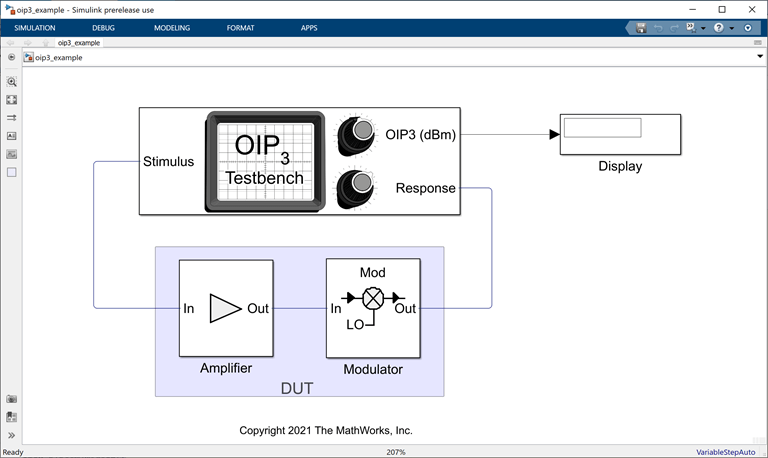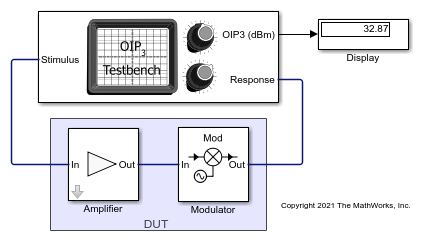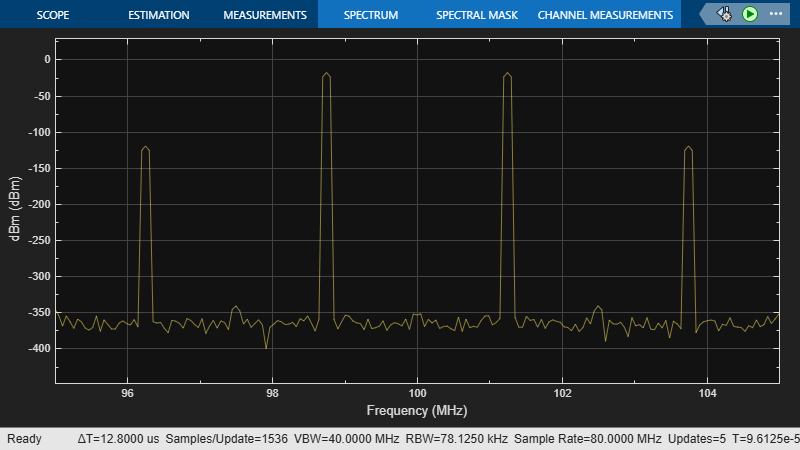Measure OIP3 of Device Under Test

Use the OIP3 Testbench block to measure the output third order intercept (OIP3) of a device under test (DUT).

Connect the blocks as shown in the model.Set the parameters for DUT and the testbench.

Amplifier block:

• Available power gain10 dB

• Intercept points conventionOutput

• IP332 dBm

Mixer block:

• Available power gain5 dB

• Local oscillator frequency2.0 GHz

• Intercept points conventionOutput

• IP335 dBm

• Filter typeHighpass

• ImplementationConstant per carrier

• Passband edge frequency2.05 GHz

OIP3 Testbench block:

• Input frequency (Hz)2.1e9

• Output frequency (Hz)0.1e9

• Simulate noise (both stimulus and DUT internal)off

Run the model. You will see that the Display block shows an OIP3 value of 32.87 dBm. This value can be verified analytically using the IIP3 equation provided in .

OIP3 of the DUT = IIP3 + Gain = – 10*log10(1/10^((22)/10) + 10^(10/10)/10^((30)/10)) + 15 = 32.8756 dBm

where,

IIP3 of the amplifier in linear scale =10^((32-10)/10), as IIP3 = OIP3 – Gain ; IIP3 = 32 dBm – 10 dB = 22 dBm

IIP3 of the mixer in linear scale = 10^((35-5)/10), as IIP3 = OIP3 – Gain ; IIP3 = 35 dBm – 5 dB = 30 dBm

Available power gain of the amplifier = 10^(10/10)Razavi, Behzad. “Basic Concepts “ in RF Microelectronics, 2nd edition, Prentice Hall, 2012.